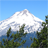# A manual example of Moran's Local I (Outlier)

1280
0
10-23-2013 04:46 AMNew Contributor
Dear forum,

I am trying to uderstand exactly how the Local Moran equation works, the equation can be found here:
http://resources.esri.com/help/9.3/arcgisdesktop/com/gp_toolref/spatial_statistics_tools/how_cluster...

For my example I have chosen a single location with a 8 cell neighbourhood, this is within a grid of 11x11 cells (121 squares), the total of all these squares is 5598 and the value of the target cell is 100.

For this cell ArcGIS gives the following results:
I-index:18,274
Z-score:6.70446
P-value: 0

I am trying to work through he equation but I am having trouble understanding all the algebra.

xi= value of the target cell = 100
X(bar) = the Average of all the values in the 121 cell grid = 46

below the division line is Si2
[
for this you take the sum of all the values but not the target cell: 5498
this is then multiplied by the result of xj minus the mean (X(bar))
xj= the neighbours?
this is then squared and divided over the number of cells (121) minus 1 = 120
the mean squared is then subtracted from this.
]

So this is Si^2

so:

100 - 46
-------------
Si^2

This is multiplied by the Sum of the neighbours in the neighbourhood (701) j not equal to i - so not including the target cell
then (xj - mean)

This gives you the I-index: Ii

this can then be used to create the Z-score as Esri indicate.

The values in the local neighbourhood for a single calculation are:

73|94|82
96|100|92
87|93|82

Can anyone help me with this?

Many thanks

Gary
Tags (2)
0 Replies